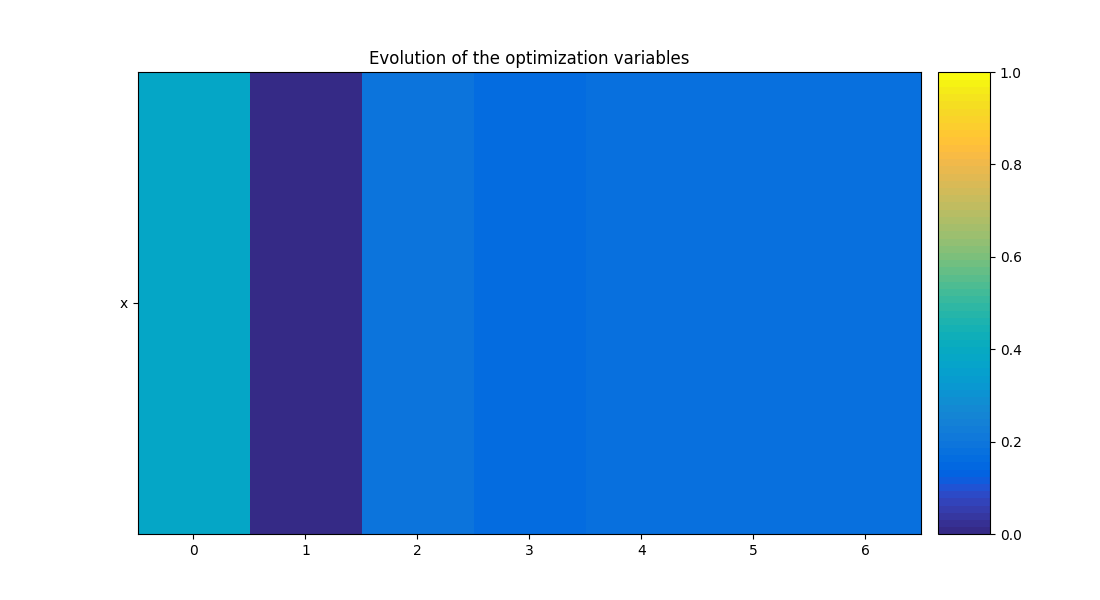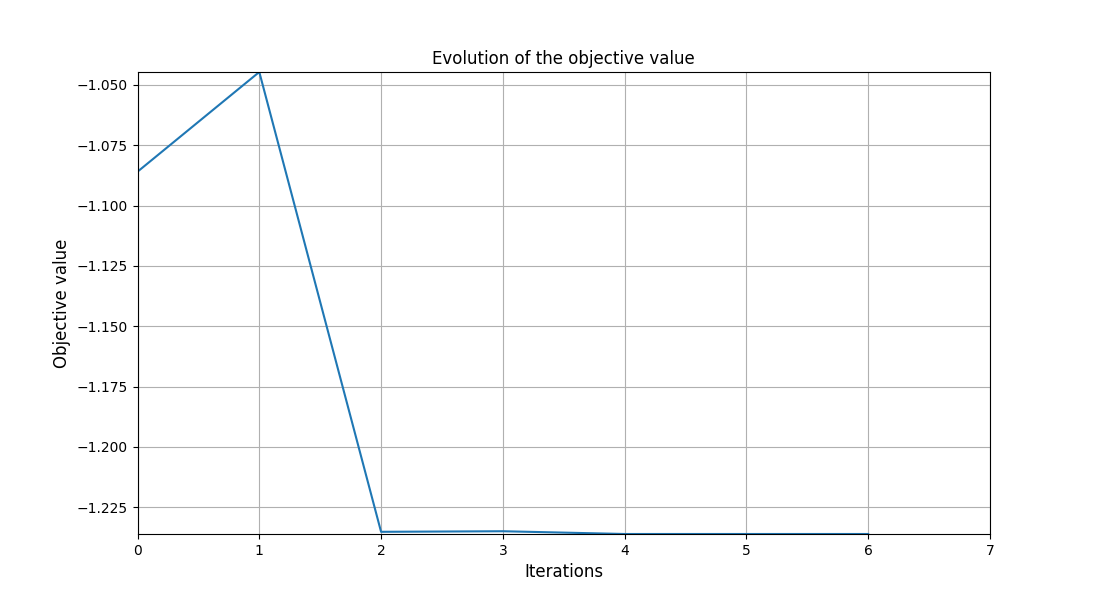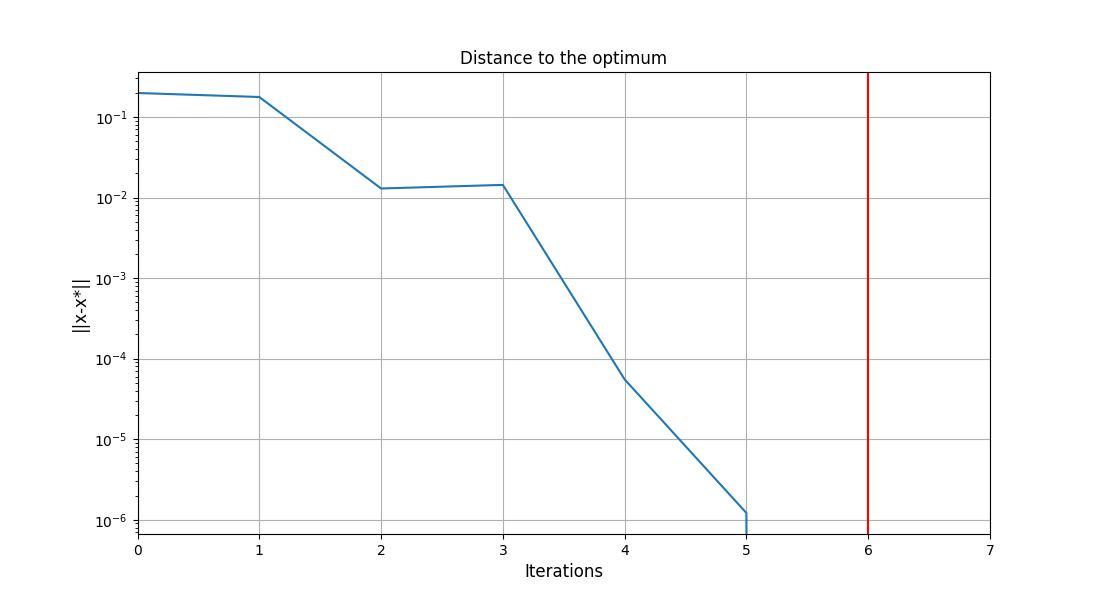# Analytical test case # 2¶

In this example, we consider a simple optimization problem to illustrate algorithms interfaces and optimization libraries integration.

## Imports¶

from __future__ import absolute_import, division, print_function, unicode_literals

from future import standard_library
from numpy import cos, exp, ones, sin

from gemseo.algos.design_space import DesignSpace
from gemseo.algos.doe.doe_factory import DOEFactory
from gemseo.algos.opt.opt_factory import OptimizersFactory
from gemseo.algos.opt_problem import OptimizationProblem
from gemseo.api import configure_logger, execute_post
from gemseo.core.function import MDOFunction

configure_logger()

standard_library.install_aliases()


## Define the objective function¶

We define the objective function $$f(x)=sin(x)-exp(x)$$ using a MDOFunction defined by the sum of MDOFunction objects.

f_1 = MDOFunction(sin, name="f_1", jac=cos, expr="sin(x)")
f_2 = MDOFunction(exp, name="f_2", jac=exp, expr="exp(x)")
objective = f_1 - f_2


The following operators are implemented: addition, subtraction and multiplication. The minus operator is also defined.

## Define the design space¶

Then, we define the DesignSpace with GEMSEO.

design_space = DesignSpace()
design_space.add_variable("x", 1, l_b=-2.0, u_b=2.0, value=-0.5 * ones(1))


## Define the optimization problem¶

Then, we define the OptimizationProblem with GEMSEO.

problem = OptimizationProblem(design_space)
problem.objective = objective


## Solve the optimization problem using an optimization algorithm¶

Finally, we solve the optimization problems with GEMSEO interface.

### Solve the problem¶

opt = OptimizersFactory().execute(problem, "L-BFGS-B", normalize_design_space=True)

print("Optimum = ", opt)


Out:

Optimum =  Optimization result:
Objective value = [-1.23610834]
The result is feasible.
Status: 0
Number of calls to the objective function by the optimizer: 8
Constraints values:


Note that you can get all the optimization algorithms names:

algo_list = OptimizersFactory().algorithms
print("Available algorithms ", algo_list)


Out:

Available algorithms  ['NLOPT_MMA', 'NLOPT_COBYLA', 'NLOPT_SLSQP', 'NLOPT_BOBYQA', 'NLOPT_BFGS', 'NLOPT_NEWUOA', 'SLSQP', 'L-BFGS-B', 'TNC']


### Save the optimization results¶

We can serialize the results for further exploitation.

problem.export_hdf("my_optim.hdf5")


### Post-process the results¶

execute_post(problem, "OptHistoryView", show=True, save=False)

•••Out:

/home/docs/checkouts/readthedocs.org/user_builds/gemseo/conda/3.0.3/lib/python3.8/site-packages/gemseo/post/opt_history_view.py:312: UserWarning: FixedFormatter should only be used together with FixedLocator
ax1.set_yticklabels(y_labels)

<gemseo.post.opt_history_view.OptHistoryView object at 0x7fc299ce4040>


Note

We can also save this plot using the arguments save=False and file_path='file_path'.

## Solve the optimization problem using a DOE algorithm¶

We can also see this optimization problem as a trade-off and solve it by means of a design of experiments (DOE).

opt = DOEFactory().execute(problem, "lhs", n_samples=10, normalize_design_space=True)
print("Optimum = ", opt)


Out:

Optimum =  Optimization result:
Objective value = [-5.34787154]
The result is feasible.
Status: None
Optimizer message: None
Number of calls to the objective function by the optimizer: 18
Constraints values:


Total running time of the script: ( 0 minutes 0.607 seconds)

Gallery generated by Sphinx-Gallery